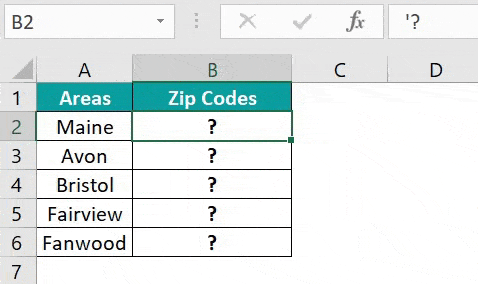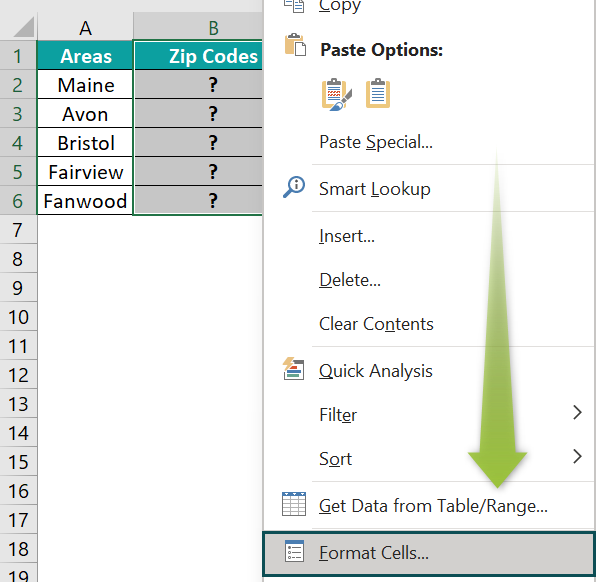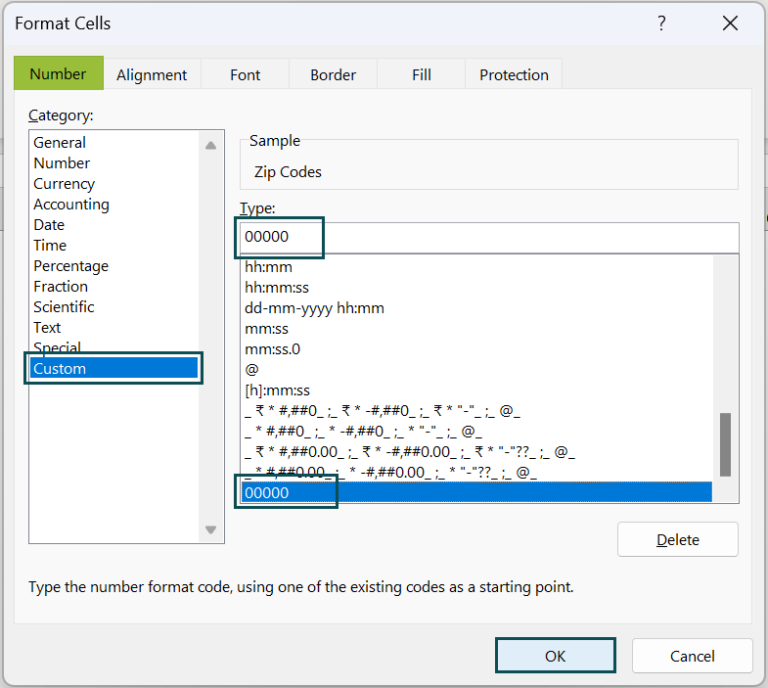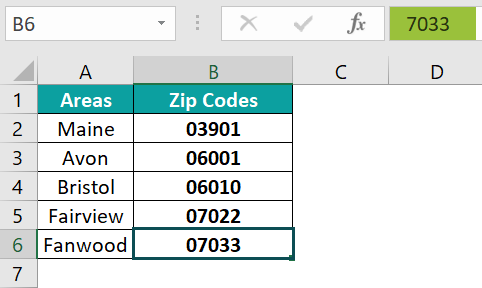Article byExcelMojo Team## What are Leading Zeros in Excel?

Whenever we enter a number with leading zeros, Excel automatically removes them. However, many numbers like product codes, zip codes, social security numbers, etc., require leading zeros. We will discuss a few methods to keep such numbers in their original format. Right from simple changes to the format to using functions like TEXT, you can maintain these leading zeros in Excel intact to store your data.

Let us look at an example of some product codes with leading zeros. To retain the zeros, we first select the cells where we want to display the number and format them as Text. For this, choose the “Text” option after clicking on the drop-down arrow in the Numbers group of the Home tab. Excel treats these cells as text and does not remove the leading zeros.

• Explanation and Uses

When you try entering a number like 001245 in Excel, it automatically trims the number by removing the leading zeros since, mathematically, leading zeros have no value.

However, there are scenarios where you need to enter numbers with leading zeros. You encounter such numbers in:

• Postal Codes
• Social Security Numbers
• Product Codes
• Phone numbers with country codes etc.

In such cases, there are many ways you can keep leading zeros in Excel as it is. Some of the different ways in which you can retain the leading zeros in Excel are as follows.

• Using the Custom Format option
• Format the cells as Text
• Using the TEXT function
• Using the RIGHT function
• Using an apostrophe

The disadvantage of some of these methods is that we can no longer use them for any mathematical calculation when the numbers get converted to Text.

###### Key Takeaways
• A number with leading zeros has the zeros removed in it automatically. To pad leading zeros, you can format the cells using the Custom format option or add an apostrophe before the numbers.
• You can also use a combination of the REPT and LEN function to add leading zeros. The leading zeros can be removed by eliminating the Custom format applied to the cells or using the VALUE function.
• We can use functions like BASE, TEXT, RIGHT, and CONCATENATE to add leading zeros. However, all these formulas convert the number to text format.

Adding leading zeros is simple and we can do it in many ways. Let us look at some of the simplest ways to do it.

1. Now, we must add the zip codes of these areas. Let us add the zip code of Maine, 03901, in B2. Press Enter. You can observe that the leading zero gets removed.2. To overcome this problem, select the cells from B1 to B6. Right-click and choose the option “Format Cells.”3. Under “Category,” choose Custom in the pop-up window.

Here, you type the required format. For example, for the zip code here, we need five digits. Hence, type five zeros as shown below.4. Press OK. Now you can observe how the leading zero is displayed. Let us also enter the other areas” zip codes.Observe how the number is displayed with leading zeros, but the formula bar does not display the zero.
Besides this, there are other methods of adding leading zeros in Excel which we will explore in the examples given below.

### Examples

#### Example #1

Below are a few numbers with preceding zeros. We must prevent Excel from removing the leading zeros. Let us look at the different methods to do it.

As seen earlier, we can show leading zeros in Excel through the Custom format or text format option. We can also use the Excel TEXT function, which pads leading zeros in cells. To insert two zeros before a number, for example, 12, we must use the formula =TEXT(cell reference, “00##”)

• Step 1: Now, let us apply the formula =TEXT(B2, 0####) in cell C2. Press Enter.
• Step 2: In the next cell, we must get four leading zeros. For this, type the following in cell C3 and press Enter.

=TEXT(B2,”000000”)

• Step 3: For cell C4, we can use the CONCATENATE excel function to add two zeros. This function is used when adding a fixed number of zeros to the value, regardless of length or type. Let us enter the following formula into cell C4 and press Enter.

=CONCATENATE(“00”,B4)

• Step 4: Since the following number also requires two preceding zeros, drag the Autofill handle to C5. Check the result.

Thus, there are many ways to add preceding zeros. The above example shows us some of the ways.

#### Example #2

Let us look at another example of using the RIGHT excel function to insert leading zeros. Here’s a list of numbers. We need to add preceding zeros but maintain the length of the numbers the same.

• Step 1: To get numbers with the same length, type the following formula in cell B2. Press Enter.

=RIGHT(“0000″&A2, 5)

Explanation:

• The first argument, “0000,” is the maximum number of zeros you can add.
• These zeros are appended by the & symbol to the value in the excel cell reference A2.
• The final argument shows the number of characters in the resulting string.
• Step 2: Drag the Autofill handle from B2 to B6. Observe how the leading zeros in Excel are added.

We can observe how the string length is maintained, and it adds the number of zeros accordingly without altering the number.

#### Example #3

Let us look at another way to add leading zeros. Below is a set of numbers. Again, we can use the less-used BASE function.

• Step 1: Let us enter the following formula in cell B2.

=BASE(A2, 10, 5)

• A2 – Cell reference of the number to be converted to the text value.
• Base – The base you want to convert the value to.
• 5– The length of the converted text value.

The above formula converts the value in cell B3 into the base and changes it to a text value with five characters. If there are less than five characters in the converted , the zeros are padded to it.

• Step 2: Now, drag the Autofill handle till B6 and check the result.

### How to Remove Leading Zeros in Excel?

1. If you have used Custom Format to add leading zeros, revert to the General format to remove leading zeros in Excel(Right click on the cell and select the option “Format Cells.”)
1. If the leading zeros were added using the apostrophe, you get a small green triangle at the top right corner.

Click on the exclamation point and click the option “Convert to Number.”

1. If a number has padded zeros added via the TEXT function, you can use the VALUE excel function to trim leading zeros in Excel.

### Important Things to Remember

• Leading zeros are significant in postal codes, social security numbers, etc. They can also be added via the Custom Format option or by formatting the cells as Text.
• We can remove leading zeros using the VALUE function for numbers converted to text.
• Also, use the Number format for numbers longer than 12 digits. Else Excel will convert it to scientific notation.

You can use the CONCATENATE function or its symbol & to add leading zeros to Excel. The format is as follows:

=CONCATENATE(“number of zeros to add”,  reference). To add zeros using &, type =”00”&(cell reference), for example, “00”&A2.

2. Why are leading zeros not showing in Excel?

In Mathematics, leading zeros in a number are considered not to have any value. So, Excel removes the leading zeros in any number we type.

To retain the zeros, we must use functions like TEXT, RIGHT, etc.

3. How to keep leading zeros in excel when combining cells?

You can use the CONCATENATE function to keep leading zeros while combining cells. For example, use the formula as follows =CONCATENATE(A2,B2)

You can also use the following formula: =A2&B2.

4. What cell format will allow for leading zeros in Excel?

To keep leading zeros in Excel, you can apply the following formatting. First, format the cells as Text in the Number group. You can also right-click, choose Format Cells, and use Custom Format.